Скачать презентацию Self consistent ion trajectories in electron shading damage

• Количество слайдов: 27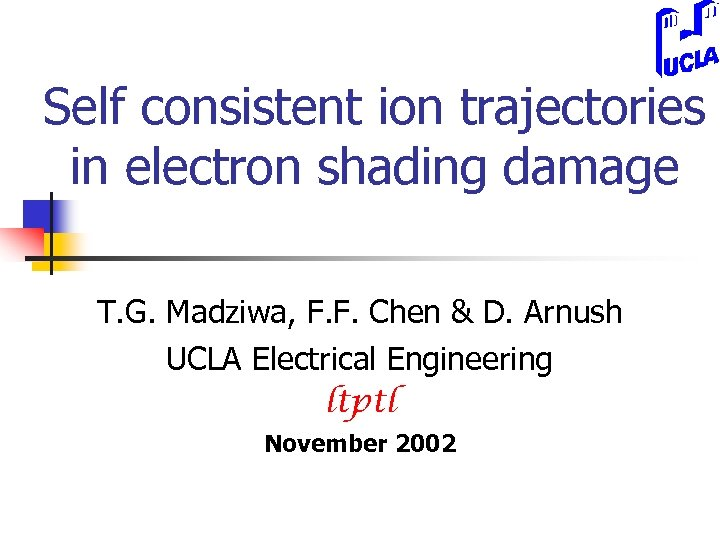Self consistent ion trajectories in electron shading damage T. G. Madziwa, F. F. Chen & D. Arnush UCLA Electrical Engineering ltptl November 2002Abstract In electron-shading damage, the photoresist is charged negatively, preventing electrons from entering the trench, while ions are accelerated toward the bottom of the trench. We have numerically calculated the effect of these fields on the ion trajectories. The ions are injected at acoustic speed from a sheath edge far from the substrate, and the electrons have a Maxwell. Boltzmann distribution. The photoresist and trench walls are assumed to be insulators, and the trench bottom a conductor at various potentials relative to the sheath edge. The potentials on all surfaces are given initial values, and a Poisson solver is used to compute the electric field everywhere. The ions’ trajectories in this field are then computed. Setting the flux of ions to each dielectric surface equal to the Maxwellian electron flux yields a new value of the surface charge. The E-fields and trajectories are then recomputed, and the process iterated until the values converge. It is found that the E-field is concentrated near the entrance to the trench, the only place where the charges matter. The ions receive a kick there and then coast the rest of the way. Thus the trajectories are very sensitive to the exact shape of the photoresist and will change as the etch progresses.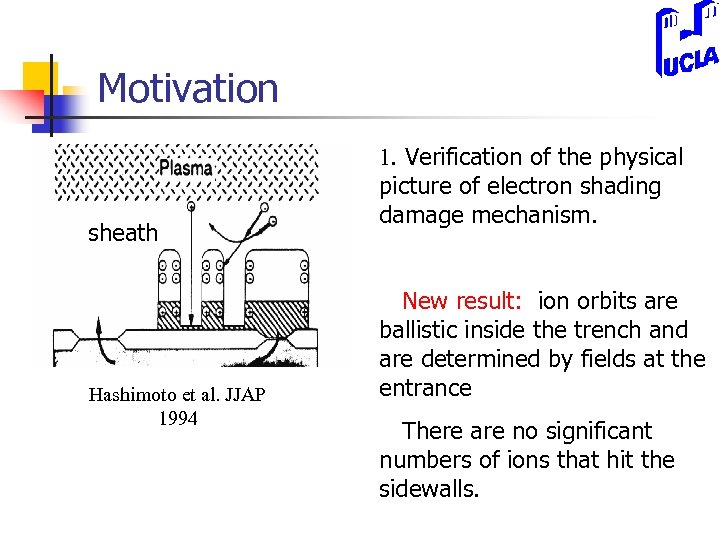Motivation sheath Hashimoto et al. JJAP 1994 1. Verification of the physical picture of electron shading damage mechanism. New result: ion orbits are ballistic inside the trench and are determined by fields at the entrance There are no significant numbers of ions that hit the sidewalls.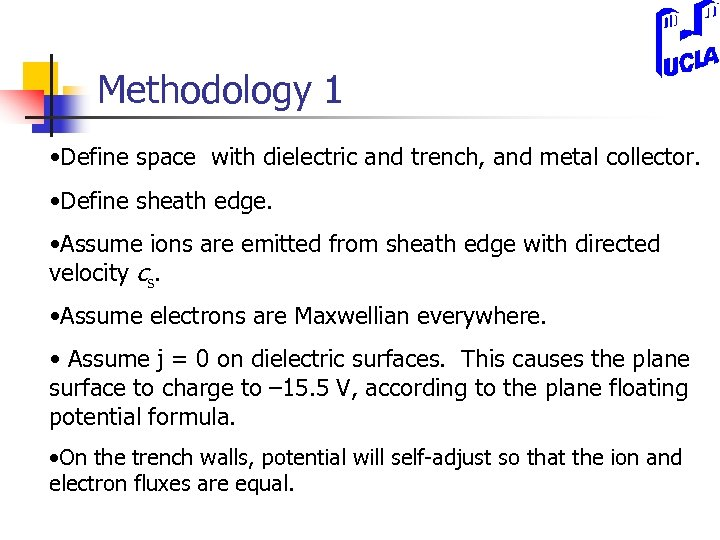Methodology 1 • Define space with dielectric and trench, and metal collector. • Define sheath edge. • Assume ions are emitted from sheath edge with directed velocity cs. • Assume electrons are Maxwellian everywhere. • Assume j = 0 on dielectric surfaces. This causes the plane surface to charge to – 15. 5 V, according to the plane floating potential formula. • On the trench walls, potential will self-adjust so that the ion and electron fluxes are equal.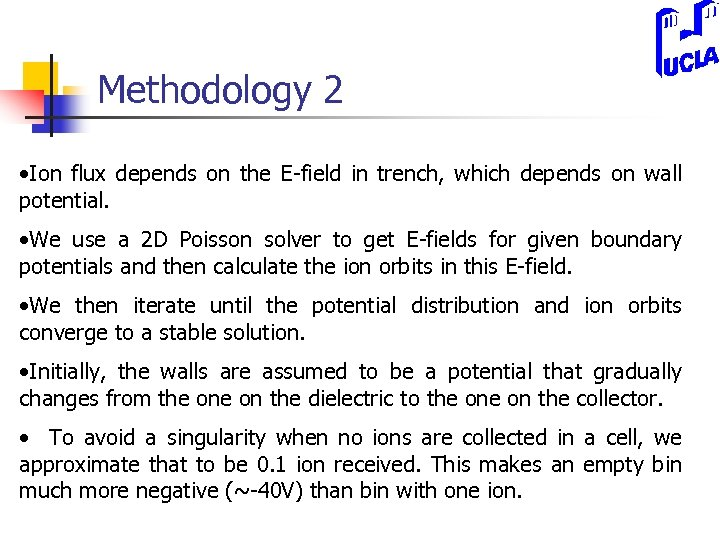Methodology 2 • Ion flux depends on the E-field in trench, which depends on wall potential. • We use a 2 D Poisson solver to get E-fields for given boundary potentials and then calculate the ion orbits in this E-field. • We then iterate until the potential distribution and ion orbits converge to a stable solution. • Initially, the walls are assumed to be a potential that gradually changes from the on the dielectric to the on the collector. • To avoid a singularity when no ions are collected in a cell, we approximate that to be 0. 1 ion received. This makes an empty bin much more negative (~-40 V) than bin with one ion.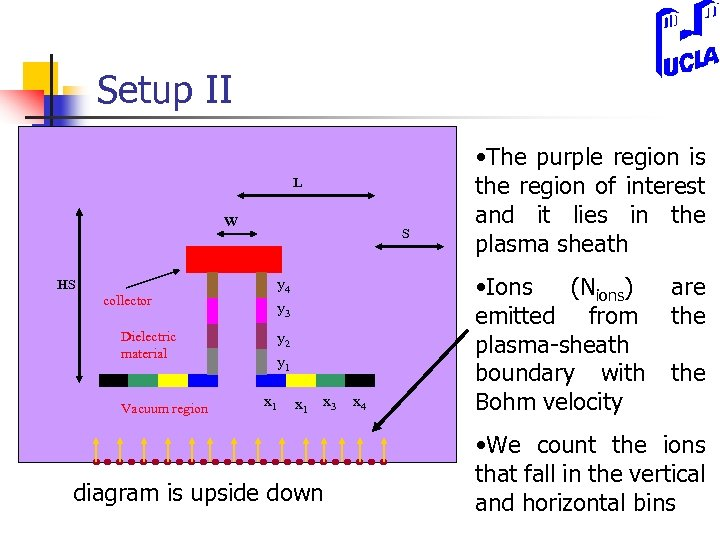Setup II L W HS collector Dielectric material Vacuum region S y 4 y 3 y 2 y 1 x 1 x 3 diagram is upside down x 4 • The purple region is the region of interest and it lies in the plasma sheath • Ions (Nions) emitted from plasma-sheath boundary with Bohm velocity are the • We count the ions that fall in the vertical and horizontal bins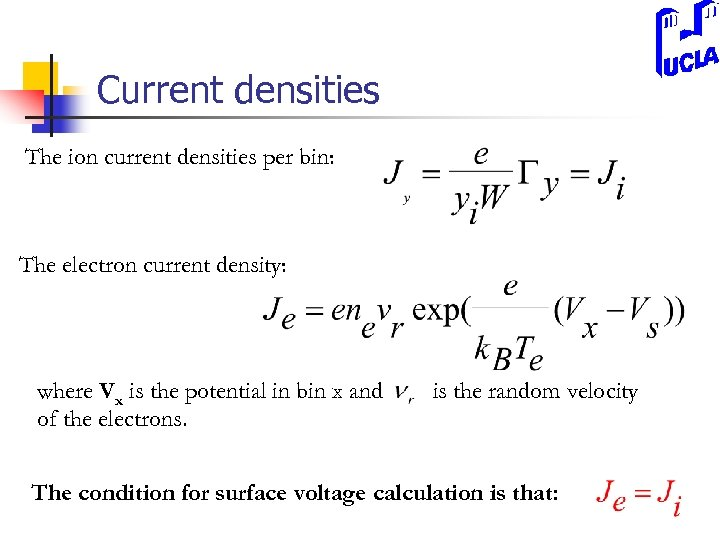Current densities The ion current densities per bin: The electron current density: where Vx is the potential in bin x and of the electrons. is the random velocity The condition for surface voltage calculation is that: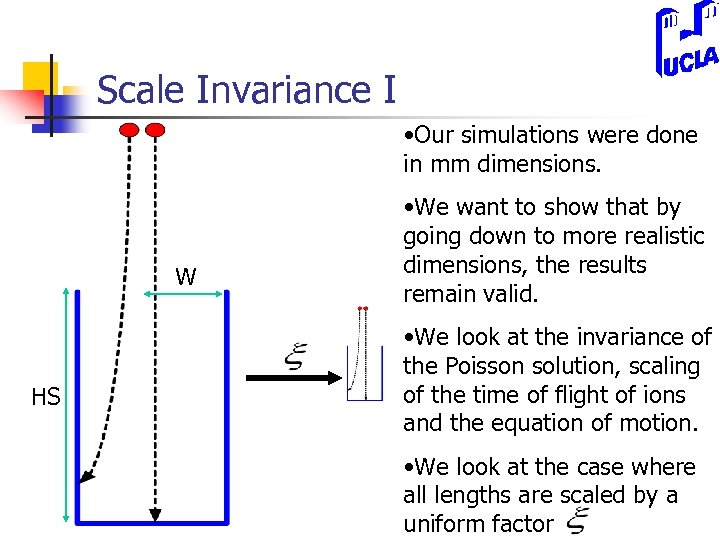Scale Invariance I • Our simulations were done in mm dimensions. W HS • We want to show that by going down to more realistic dimensions, the results remain valid. • We look at the invariance of the Poisson solution, scaling of the time of flight of ions and the equation of motion. • We look at the case where all lengths are scaled by a uniform factor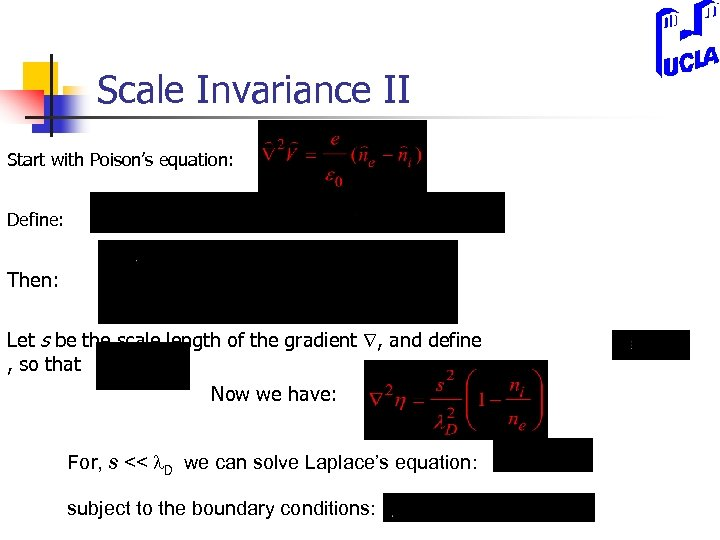Scale Invariance II Start with Poison’s equation: Define: Then: Let s be the scale length of the gradient , and define , so that Now we have: For, s << l. D we can solve Laplace’s equation: subject to the boundary conditions: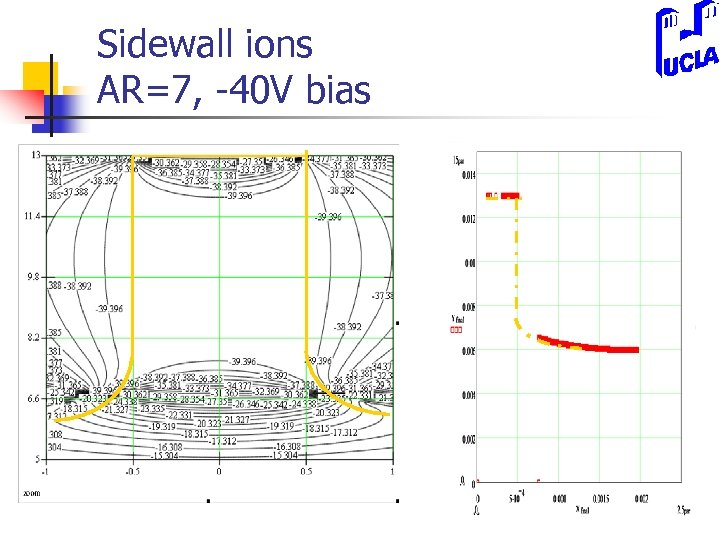Sidewall ions AR=7, -40 V bias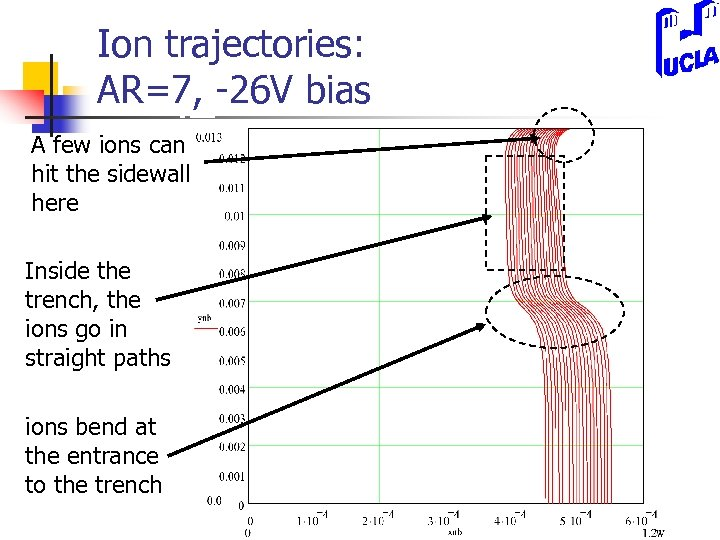Ion trajectories: AR=7, -26 V bias A few ions can hit the sidewall here Inside the trench, the ions go in straight paths ions bend at the entrance to the trench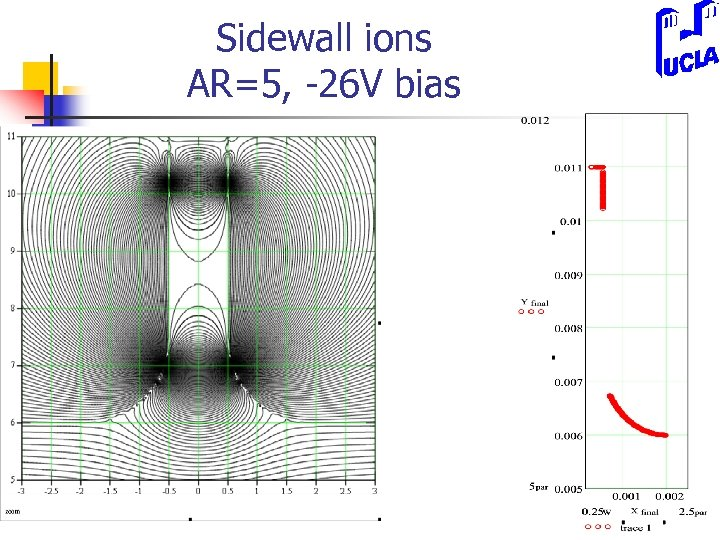Sidewall ions AR=5, -26 V bias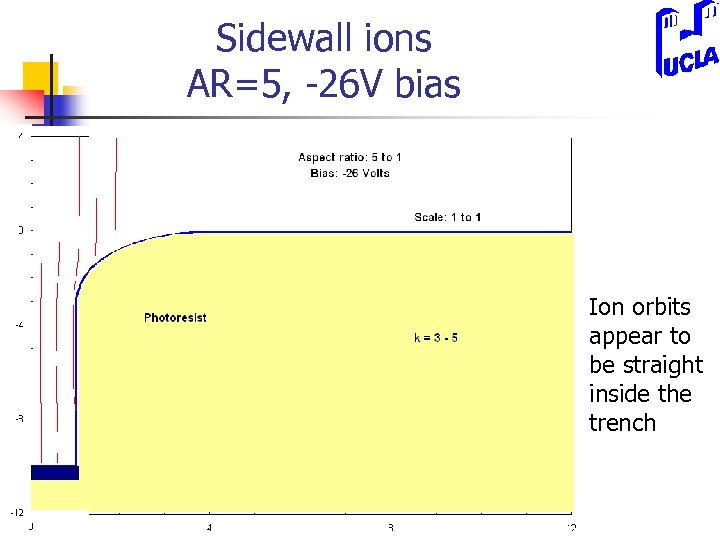Sidewall ions AR=5, -26 V bias Ion orbits appear to be straight inside the trench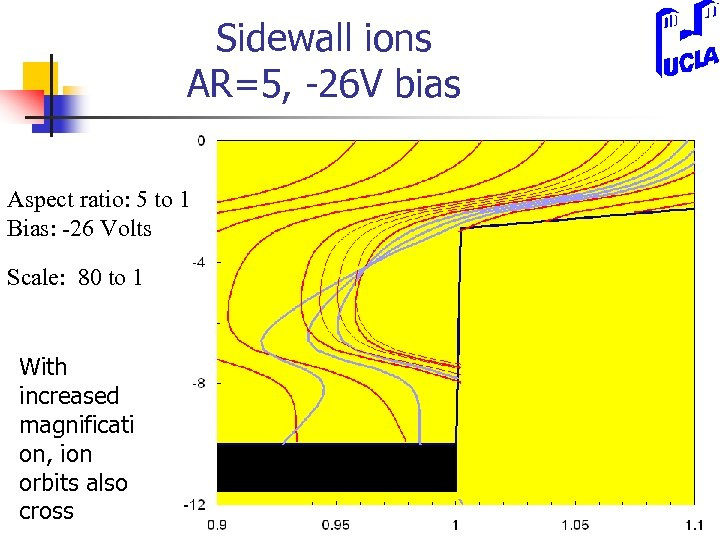Sidewall ions AR=5, -26 V bias Aspect ratio: 5 to 1 Bias: -26 Volts Scale: 80 to 1 With increased magnificati on, ion orbits also cross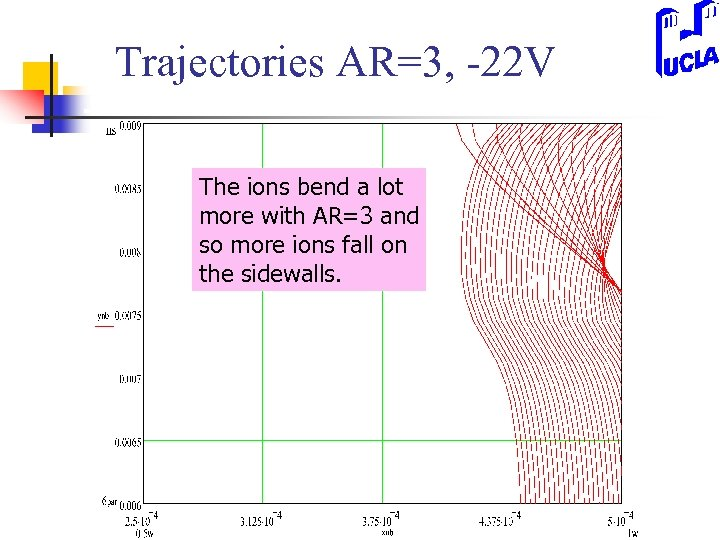Trajectories AR=3, -22 V The ions bend a lot more with AR=3 and so more ions fall on the sidewalls.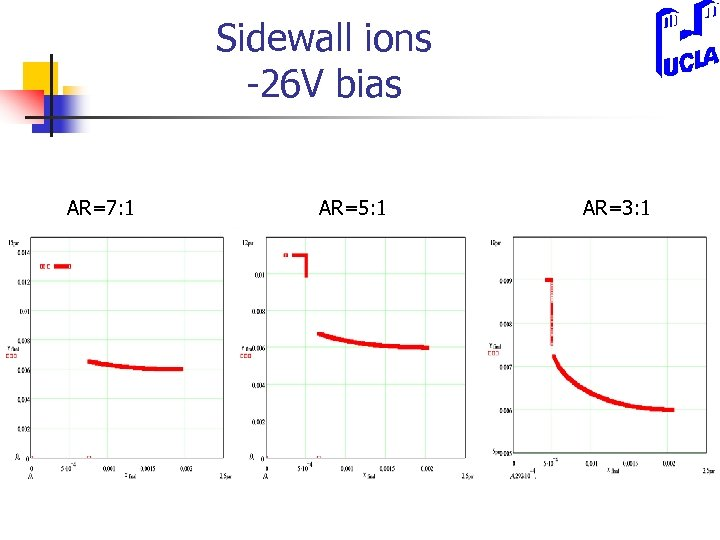Sidewall ions -26 V bias AR=7: 1 AR=5: 1 AR=3: 1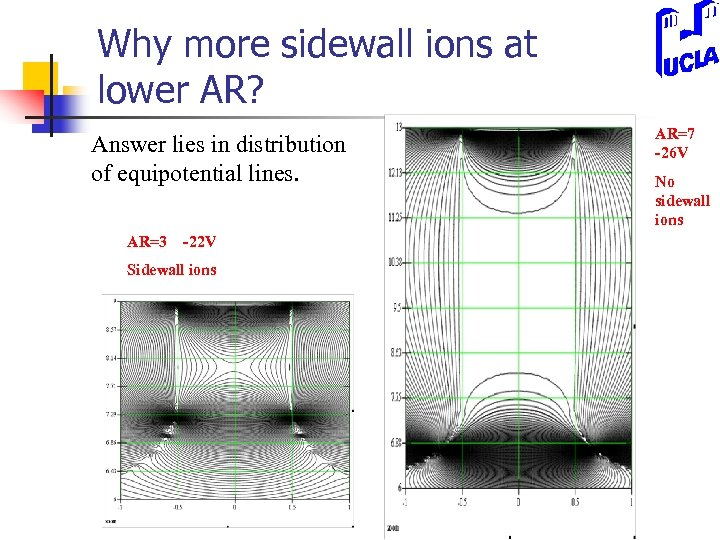Why more sidewall ions at lower AR? Answer lies in distribution of equipotential lines. AR=3 -22 V Sidewall ions AR=7 -26 V No sidewall ions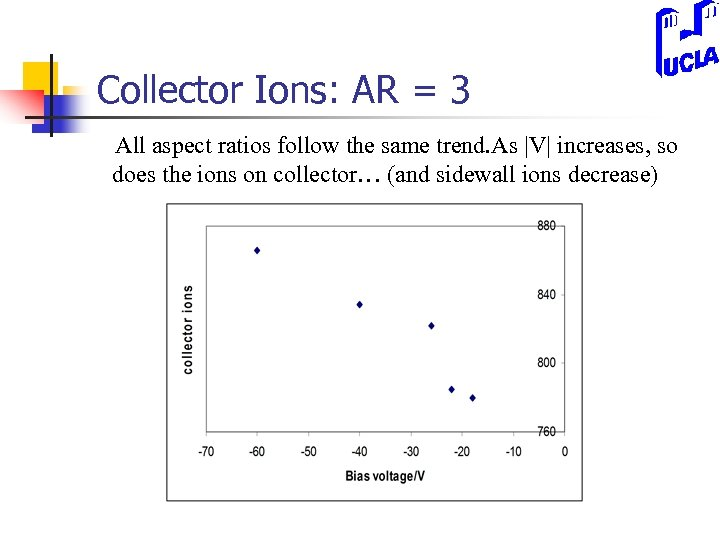Collector Ions: AR = 3 All aspect ratios follow the same trend. As |V| increases, so does the ions on collector… (and sidewall ions decrease)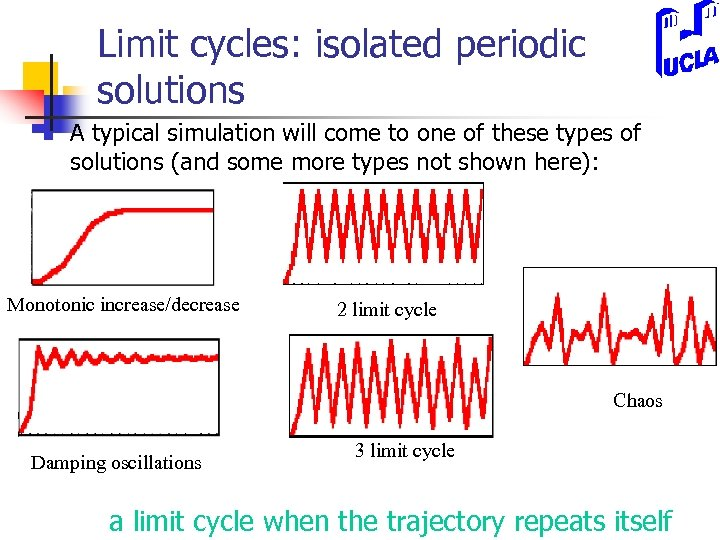Limit cycles: isolated periodic solutions n A typical simulation will come to one of these types of solutions (and some more types not shown here): Monotonic increase/decrease 2 limit cycle Chaos Damping oscillations 3 limit cycle a limit cycle when the trajectory repeats itself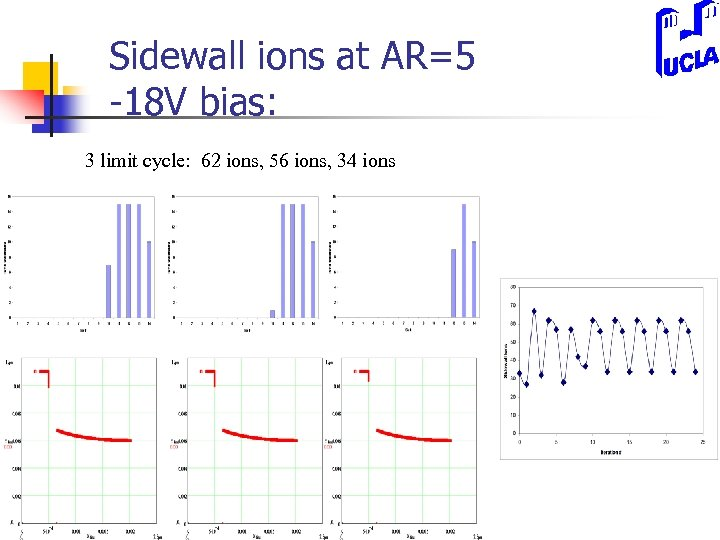Sidewall ions at AR=5 -18 V bias: 3 limit cycle: 62 ions, 56 ions, 34 ions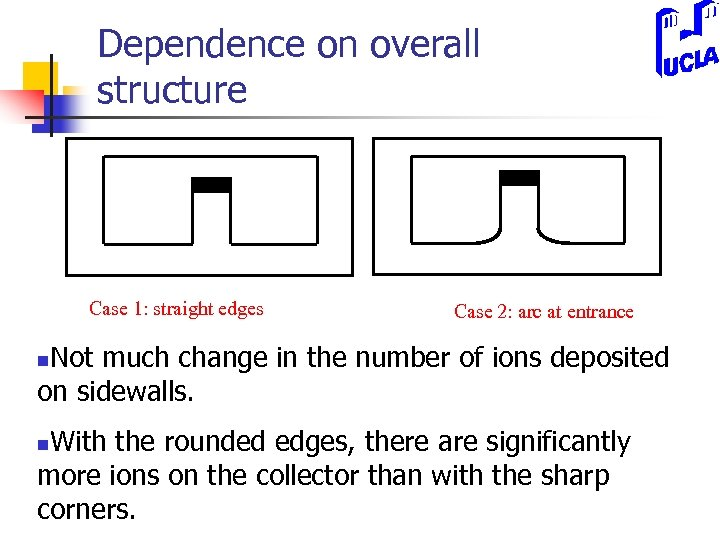Dependence on overall structure Case 1: straight edges Case 2: arc at entrance Not much change in the number of ions deposited on sidewalls. n With the rounded edges, there are significantly more ions on the collector than with the sharp corners. n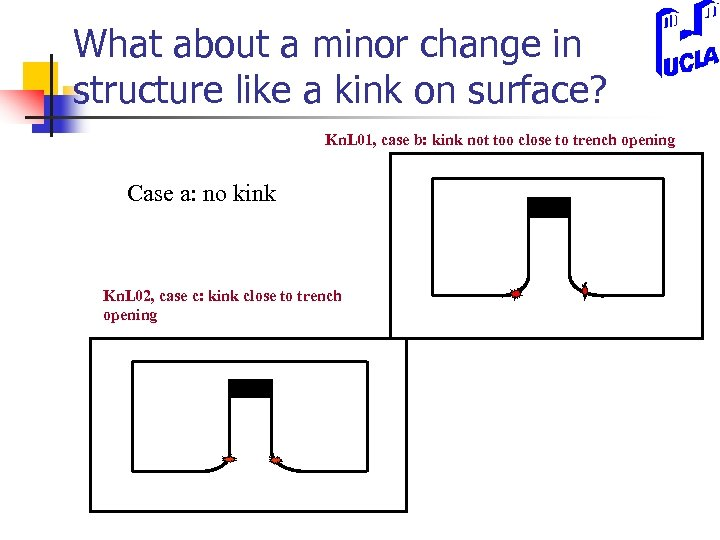What about a minor change in structure like a kink on surface? Kn. L 01, case b: kink not too close to trench opening Case a: no kink Kn. L 02, case c: kink close to trench opening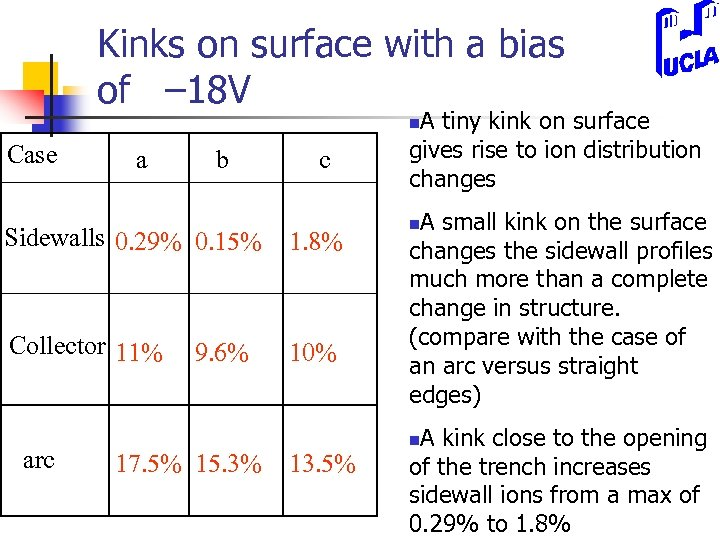Kinks on surface with a bias of – 18 V A tiny kink on surface gives rise to ion distribution changes n Case a b c Sidewalls 0. 29% 0. 15% 1. 8% Collector 11% 10% arc 9. 6% 17. 5% 15. 3% 13. 5% A small kink on the surface changes the sidewall profiles much more than a complete change in structure. (compare with the case of an arc versus straight edges) n A kink close to the opening of the trench increases sidewall ions from a max of 0. 29% to 1. 8% n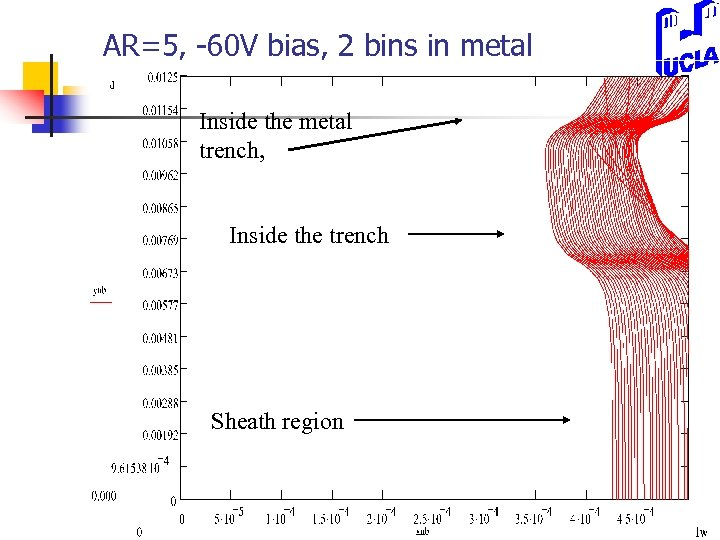AR=5, -60 V bias, 2 bins in metal Inside the metal trench, Inside the trench Sheath region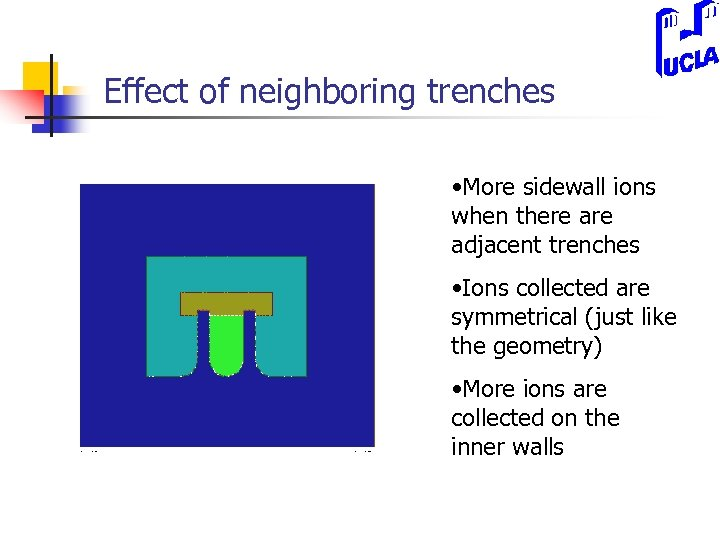Effect of neighboring trenches • More sidewall ions when there adjacent trenches • Ions collected are symmetrical (just like the geometry) • More ions are collected on the inner walls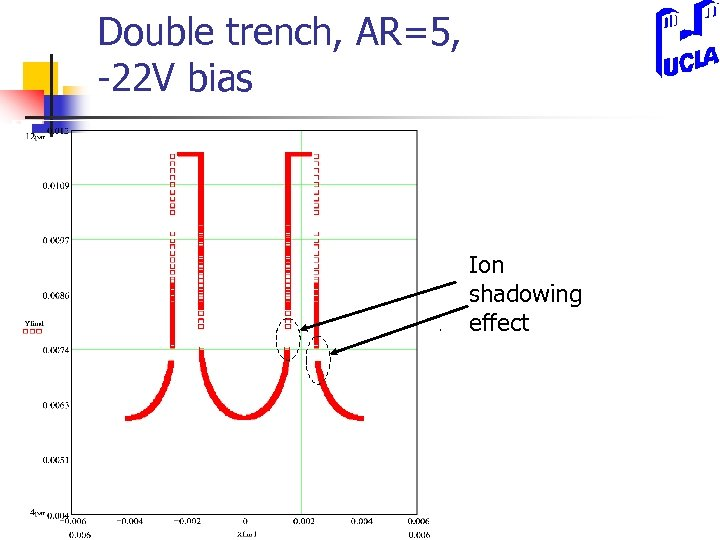Double trench, AR=5, -22 V bias Ion shadowing effect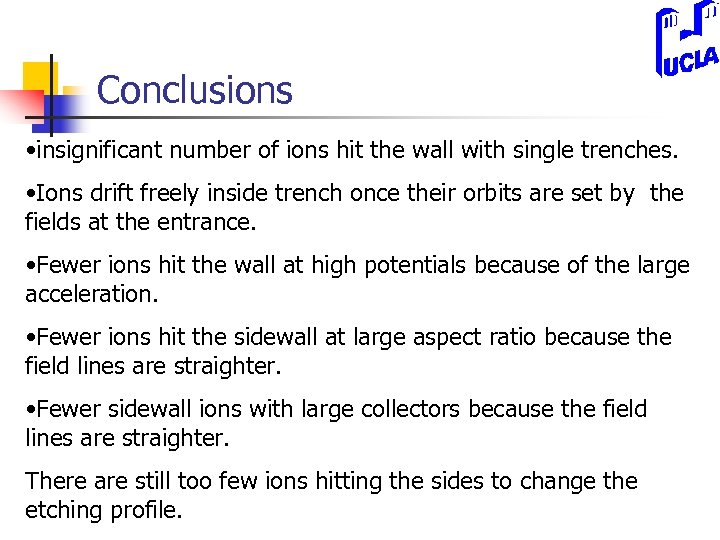Conclusions • insignificant number of ions hit the wall with single trenches. • Ions drift freely inside trench once their orbits are set by the fields at the entrance. • Fewer ions hit the wall at high potentials because of the large acceleration. • Fewer ions hit the sidewall at large aspect ratio because the field lines are straighter. • Fewer sidewall ions with large collectors because the field lines are straighter. There are still too few ions hitting the sides to change the etching profile.